# Quadratic formula to find n^(th) term of a sequence easier method - consistent difference between difference

## Method 2

This method is trickier to remember but less work to find an answer.

We know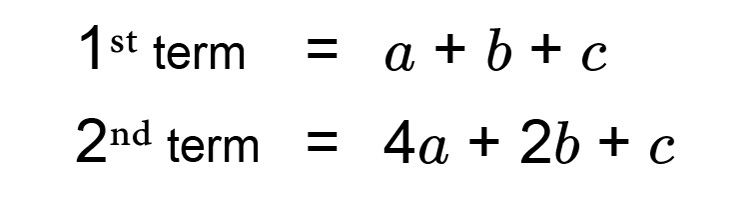and mathematicians also found that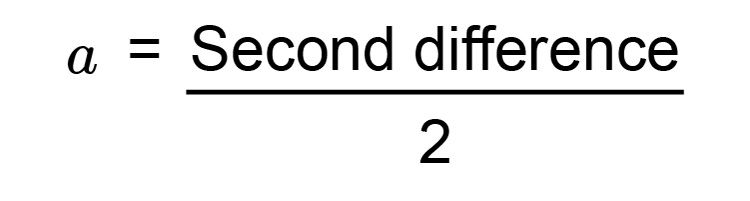Once you have found a,\ b,\ &\ c you can slot these figures in the following formula

an^2+bn+c

Example 1

We know the following sequence is a quadratic sequence but what is the formula for the n^(th) term using an easier method?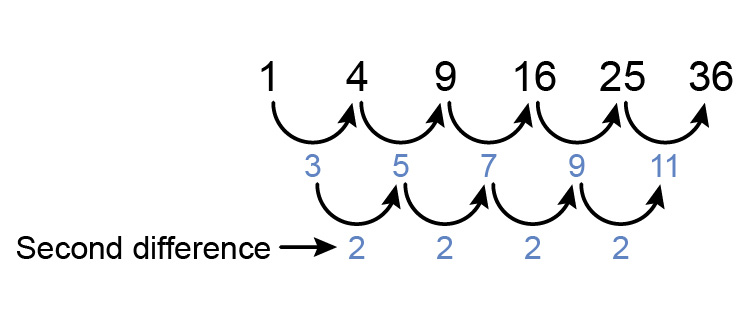The 1st term =a+b+c

so                 1=a+b+c

The 2nd term =4a+2b+c

so                   4=4a+2b+c

and a=(se\cond\ \ di\fference)/2

a=2/2=1

Now we have

1=a+b+c  ....................  (1)

4=4a+2b+c  ................  (2)

and a=1

therefore equation (1) becomes  1=1+b+c

1-1=b+c

-b=c

therefore equation (2) becomes  4=4times1+2b-b

4-4=2b-b

0=2b-b

0=1b

b=0

Now substitute a=1 and b=0 into equation (1)

1=a+b+c

1=1+0+c

c=1-1=0

Summary a=1   b=0   and   c=0

therefore the formula for the n^(th)  term in this sequence is

an^2+bn+c

or   1n^2+0n+0

which is        n^2

Now we need to check the formula is correct.

Try different values of n in the formula n^2

If  n=1       term  =1^2=1

If  n=2       term  =2^2=4

If  n=3       term  =3^2=9

If  n=4       term  =4^2=16

This is correct

Answer =n^2

Example 2

We know the following sequence is a quadratic sequence but what is the formula for the n^(th) term using an easier method?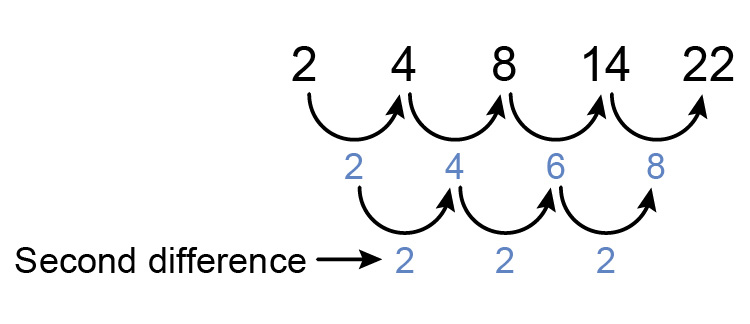The 1st term =a+b+c

so                 2=a+b+c

The 2nd term =4a+2b+c

so                   4=4a+2b+c

and  a=(se\cond\ \ di\fference)/2

a=2/2=1

Now we have

2=a+b+c  ....................  (1)

4=4a+2b+c  ................  (2)

and a=1

therefore equation (1) becomes 2=1+b+c

1=b+c........... (i)

therefore equation (2) becomes 4=4times1+2b+c

4=4+2b+c

4-4=2b+c

0=2b+c ......... (ii)

Now we have two simultaneous equations:

1=b+c  ...........  (i)

0=2b+c  .........  (ii)

From (i) we get b=1-c

Substitute that into (ii) we get

0=2(1-c)+c

0=2-2c+c

0=2-1c

-2=-c

c=2

Now substitute c=2 into (i)

1=b+2

b=1-2

b=-1

Summary a=1   b=-1   and   c=2

therefore the formula for the n^(th)  term in this sequence is

an^2+bn+c

or   1n^2+(-1)n+2

which is        n^2-n+2

Now we need to check the formula is correct.

Try different values of n in the formula n^2-n+2

If  n=1       term  =1^2-1+2=2

If  n=2       term  =2^2-2+2=4-2+2=4

If  n=3       term  =3^2-3+2=9-3+2=8

If  n=4       term  =4^2-4+2=16-4+2=14

This is correct

Example 3

We know the following sequence is a quadratic sequence but what is the formula for the n^(th) term using an easier method?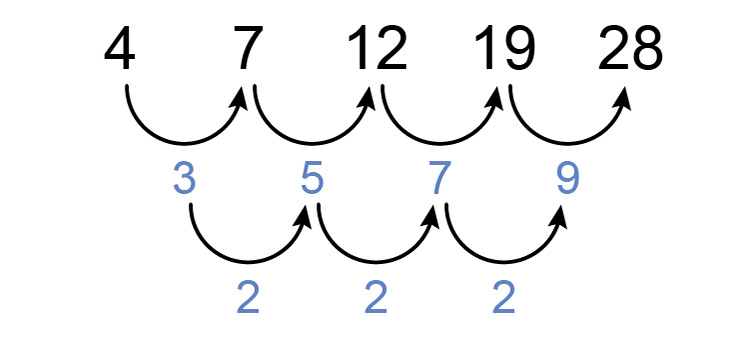The 1st term =a+b+c

so                 4=a+b+c

The 2nd term =4a+2b+c

so                   7=4a+2b+c

and  a=(se\cond\ \ di\fference)/2

a=2/2=1

Now we have

4=a+b+c  ...................  (1)

7=4a+2b+c  ..............  (2)

and a=1

therefore equation (1) becomes 4=1+b+c

3=b+c........... (i)

therefore equation (2) becomes  7=4times1+2b+c

7=4+2b+c

7-4=2b+c

3=2b+c ......... (ii)

Now we have two simultaneous equations:

3=b+c  ...........  (i)

3=2b+c  .........  (ii)

From (i) we get b=3-c

Substitute that into (ii) we get

3=2(3-c)+c

3=6-2c+c

3-6=-2c+c

-3=-c

c=-3/-1=3

Now substitute c=3 into (i)

3=b+3

b=3-3

b=0

Summary a=1   b=0   and   c=3

therefore the formula for the n^(th)  term in this sequence is

an^2+bn+c

or   1n^2+0n+3

which is         n^2+3

Now we need to check the formula is correct.

Try different values of n in the formula n^2+3

If  n=1       term  =1^2+3=4

If  n=2       term  =2^2+3=4+3=7

If  n=3       term  =3^2+3=9+3=12

If  n=4       term  =4^2+3=16+3=19

This is correct

Answer n^2+3

Example 4

We know the following sequence is a quadratic sequence but what is the formula for the n^(th) term using an easier method?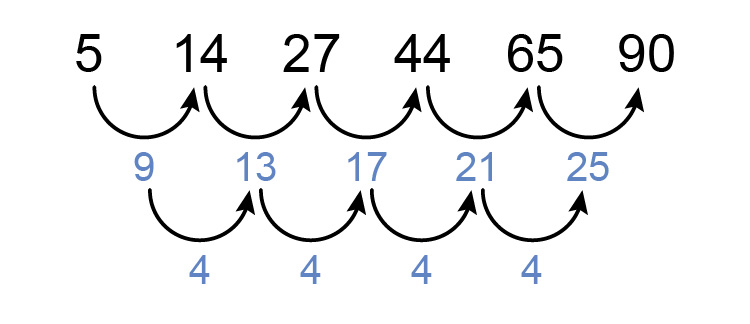The 1st term =a+b+c

so                 5=a+b+c

The 2nd term =4a+2b+c

so                14=4a+2b+c

and  a=(se\cond\ \ di\fference)/2

a=4/2=2

Now we have

5=a+b+c  ....................  (1)

14=4a+2b+c  ................  (2)

and a=2

therefore equation (1) becomes 5=2+b+c

3=b+c........... (i)

therefore equation (2) becomes 14=4times2+2b+c

14=8+2b+c

6=2b+c ......... (ii)

Now we have two simultaneous equations:

3=b+c  ...........  (i)

6=2b+c  .........  (ii)

From (i) we get b=3-c

Substitute that into (ii) we get

6=2(3-c)+c

6=6-2c+c

6-6=-2c+c

0=-c

c=0

Now substitute c=0 into (i)

3=b+c

3=b+0

b=3

Summary a=2   b=3   and   c=0

therefore the formula for the n^(th)  term in this sequence is

an^2+bn+c

or   2n^2+3n+0

which is        2n^2+3n

Now we need to check the formula is correct.

Try different values of n in the formula 2n^2+3n

If  n=1       term  =2times1^2+3times1=2+3=5

If  n=2       term  =2times2^2+3times2=8+6=14

If  n=3       term  =2times3^2+3times3=18+9=27

If  n=4       term  =2times4^2+3times4=32+12=44

This is correct

Answer 2n^2+3n